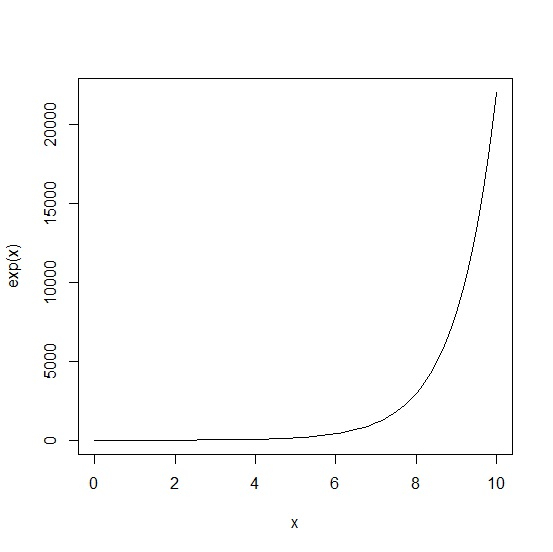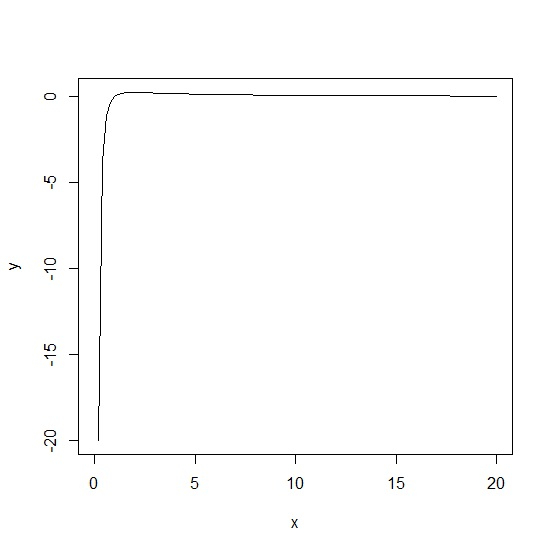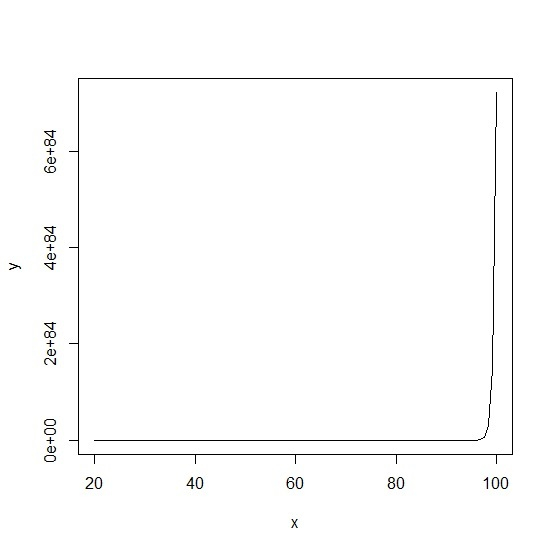# How to plot a function in R?

Plotting a function in R is not a difficult task. We can do it simply with curve function but if the function is very complex then it inside curve function might be difficult. It totally depends on the understand of the person who wants to plot the function, if he or she is well versed with the function then it won’t take much time, otherwise it becomes tedious.

## Example

> curve(exp(x),from=0, to=10)

## Output> curve((x-1)/(x^2),from=0, to=20,ylab="y")

## Output> curve(((exp(x))^2)/(x),from=20, to=100,ylab="y")

## Output